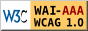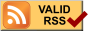# 高等演算法-計算幾何(Advanced Algorithm-Computational Geometry)

## 簡介

Cobham 和Eomond 首先指出，不同的演算法有不同的時間複雜度(time complexity)，一些針對well-defined 問題所發展出來的演算法，有些屬於「多項式時間演算法」(polynomial time algorithms)，有些則是「指數時間演算法」(exponential time algorithms)。對於後者，當問題的複雜度加大時，會使得該些演算法的演算時間呈指數成長，導致求得解答所需的時間可能需花費數年，甚至數世紀或更長時間。之後，Cook 提出「NP-complete 理論」，從此證實了運用資訊科技演算法求解也存在時間限制。

• 資料結構-圖
• 離散數學-集合與函數
• 演算法
• 幾何學
• 拓樸學
• 多維空間

## Asymptotic Notations

### O的比較

1. 小時候胖不是胖原則：眼光要放遠，我們在乎的是n很大的時候。
2. 方格紙y軸可以均勻縮放原則:

### 正式的定義

• O(f(n)):所有成長速率相等慢於f(n)的函數所成之集合(<=的概念) 大O
• Ω(f(n)):所有成長速率相等快於f(n)的函數所成之集合(>=的概念) 大OMEGA
• θ(f(n)):所有成長速率相等於f(n)的函數所成之集合(=的概念) 大theta
• o(f(n)):所有成長速率慢於f(n)的函數所成之集合(<的概念) 小O
• ω(f(n)):所有成長速率快於f(n)的函數所成之集合(>的概念) 小omega

big o :Ο

omega:Ω

theta:θ

### 函數與數字的比較

θ vs. =
 θ = transitivity(遞移性) f() a=b ^ b=c ==> a=c reflexivity(反射性) a=a symmetry(對稱性) a=b ==> b=a
O vs. <=
O <= transitivity(遞移性) a<=b ^ b<=c ==> a<=c reflexivity(反射性) a<=a anti-symmetry(反對稱性) a<=b ^ b<=a ==> a=b
Ω vs. >=
Ω >= transitivity(遞移性) a>=b ^ b>=c ==> a>=c reflexivity(反射性) a>=a anti-symmetry(反對稱性) a>=b ^ b>=a ==> a=b
o vs. <
o < transitivity(遞移性) a a
ω vs. >
ω > transitivity(遞移性) a>b ^ b>c ==> a>c

branch search

## 幾何觀念(Geometry Concept)

• 點(Points) = 0-flat = 端點(vertex)
• 線(Lines) = 1-flat = 邊(edge)
• 面(Planes) = 2-flat
• 體() = 3-flat
• 球(Spheres)
• 向量(Vector)
• 內積(inner product)
• 外積(cross product)
• 凸集合(Convex Set)

## 計算幾何(Computational Geometry;CG)

• 凸多邊型(Convex hull)
• 交點問題(Intersection Problem)：包含了物件或線段的交點偵測或避免，大多用在機械人(Robotics)
• 線段的交點(Line Segment Intersection)
• 平面、多邊形與藝廊問題(Planar Graphs,Polygons and Art Galleries)
• 切線與最短路徑(Triangulation & Shortest Paths)
• Range Searching
• Point Location
• Voronoi Diagrams
• Delaunay Triangulation
• Arrangements
• Combinatorial Geometry
• Epsilon Nets and VC Dimension
• Some paradoxes of Higher Dimension
• Closet Pair Problem
• Binary Space Partitions

### 凸多邊型(Convex Hull;CH)

#### 一般位置假設(General Position Assumption;GPA)：3點不共線

• 由reduction可知CH與sort問題關係密切
• 在f(n)的時間內可將sort問題簡化為CH的問題，所以sort問題不會比CH更難
• 何謂input sensitive與output sensitive
• 寫一個不考慮演算法的O(n^2) 的虛擬碼
• CH的各種演算法與sort的關係與其時間複雜度,特性
• Graham scan
• Jarvis march
• Divide & Conqer
• Quick
• Chans's
• Chans's algorithm的support線段副程式的虛擬碼
• 在CH上的GPA為何?
• CH上演算法的極限Ω(n㏒n)

### 線段的交點(Line Segment Intersection)

• 在平面上給n條線段，求每2條線段的交點
• 線段僅給予2個端點的座標
• n個線段可能有0個到n^2個交點,假設有I個交點
• 最一般的演算法，就是比較所有的線段是否有交點，時間複雜度為O(n^2)
• 希望找到一個output sensitive的演算法，理想的時間複雜度為O(n㏒n + I)

1. 沒有垂直的線段
2. 2條線段會交於一點而沒有平行的狀況
3. 沒有3條線段交於一點的情況

1. 給予2條線段座標a(1,2) b(3,4) c(2,4) d(4,2)
1. 根據內積公式ax*by-bx*ay, 我們以線段ab為準
2. |3 4| 與 |3 4| 得出 3*4-2*4=4 與 3*2-4*4=-10
|2 4|　　|4 2|
3. 一正一負代表2線段有相交
2. 給予1線段a(1,2) b(3,4)，一直線方程式x+y-6=0
1. 將a座標帶入直線方程式中=>1+2-6<0
2. 將b座標帶入直線方程式中=>3+4-6>0
3. 一正一負代表2線段有相交

1. 給予2條線段座標a(1,2) b(3,4) c(2,4) d(4,2) 經上式計算已知有相交，求交點
2. 根據convex combination {(x,y)|(x,y)=λ(x1y1)+(1-λ)(x2,y2),for some λ=[0,1]}
線段ab的公式為s*a + (1-s)*b
線段cd的公式為t*c + (1-t)*d
3. 因為我們知道線段ab與線段cd會有交點,所以(s=t)
x= s*ax + (1-s)*bx = t*cx + (1-t)*dx => s + (1-s)*3 = 2t + (1-t)*4 => 3-2s = 4-2t => 2s-2t=-1 .....Ⅰ
y= s*ay + (1-s)*by = t*cy + (1-t)*dy => 2s + (1-s)*4 = 4t + (2-2t)*2 => 4-2s = 2+2t => 2s+2t=2 ....Ⅱ
4. 將第-式求得t=0.75,帶入第式求得s=0.25,將s代入上式求得交點(2.5,3.5)

1. 公式為 => (Y-Y1) / (Y2-Y1) = (X-X1) / (X2-X1)
2. 給予線段座標 (2,4) (4,2)
3. 代入公式 => (y-4) / (2-4) = (x-2) / (4-2) => -y+4 = x-2
4. x+y=6

#### Plane Sweep Algorithm

• 掃瞄線狀態(Sweep Line Status):由上而下有順序的存放掃瞄到的線段名稱
• 以平衡二元樹儲存，以目前掃瞄到線段y座標當索引值
• 插入刪除搜尋的時間複雜度為O(㏒n)
• 遇到事件時以其x座標計算所有在sweep line status中的線段y座標
• 事件佇列(Event Queue):以x座標記錄所有線段的端點，與線段的交點
• 以平衡二元樹儲存，x座標排序為其索引
• 當掃瞄線遇到線段的開始，結束或交點時才會變動其值
• 線段的端點是在一開始就加入的，但線段的交點是動態加入的

1. 將所有的端點資料依x座標排序輸入event queue, sweep line status一開始是空的
2. 當enent queue不是空的,從queue中取出下一個event
1. 如果是線段左邊的端點
1. 將這個線段名稱加入sweep line status,並依y座標排序
2.
與上下2個線段計算有無交點,若有交點則依其x座標加入enent queue
2. 如果是線段右邊的端點
1. 從sweep line status刪除其線段名稱
2. 刪除後的上下線段則再計算有無交點,若有交點則依其x座標加入enent queue
3. 如果遇到交點
1. 對調在sweep line status中的線段位置
2. 重新計算上下2條線段與其鄰近的線段有無交點,若有交點則依其x座標加入enent queue

### 平面、多邊形與藝廊問題(Planar Graphs,Polygons and Art Galleries)

#### 平面圖(Planar Graph)上的特性：尤拉公式(Euler's formula)

• 完全連接圖：V-E+F=2 //端點(Vertex)-邊(Edge)+面(Face)=2
• 不完全連接圖：V-E+F-C=1//端點(Vertex)-邊(Edge)+面(Face)-子圖的個數(Component)=1
• 證明：已知頂點數，則E,F不會太多
• 已知：E=(F*3)/2 //每個面有3個邊
• 代入尤拉公式推演後：
• V=2+(F/2)
• E=3V-6或E=3/2F
• F=2V-4

#### 多邊形曲線(Polygonal Curves)

simple polygons 若有n個頂點則有n-3個對角線(diagonals)，有n-2個三角形(triangles)

#### 藝廊問題(Art Gallery)

• 一個藝廊的平面圖為一個單純多邊形，要多少個警衛才能看到整個房間
• 每個警衛為固定不可移動眼界可360度環繞但不可穿透牆壁

### 將多邊型切割成三角形(Polygon Triangulation)

#### 簡介

• 一個多邊型要被描述通常將它們切割成多個三角形後會較好處理，例如計算面積等
• 計算幾何的問題之所以困難是因為結合了組合(combinatorial)與幾何(geometrical)的議題，而將多邊型切割成許多三角形就可以去除其中的幾何困擾了。

• 直徑(diameter)
• 弧(arc)

## 網路資源|||||